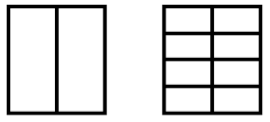# Addition and Subtraction of Fractions/Decimals

## Objective

Find equivalent fractions.

## Common Core Standards

### Core Standards

?

• 5.NF.A — Use equivalent fractions as a strategy to add and subtract fractions.

?

• 4.NF.A.1

## Criteria for Success

?

1. Understand that equivalent fractions are fractions of the same size or at the same point on a number line.
2. Generate equivalent fractions using an area model, a number line, or multiplication/division (MP.5).
3. Determine whether two fractions are equivalent using an area model, a number line, or multiplication/division (MP.3).

## Tips for Teachers

?

• Lesson 1 is intended to address the cluster heading “use equivalent fractions as a strategy to add and subtract fractions” (5.NF.A) by ensuring that students are able to recognize and generate equivalent fractions. Recognizing and generating equivalent fractions is an expectation in Grade 4 (4.NF.1), so it is up to your discretion about whether this lesson is needed or not. But, since using equivalent fractions to add and subtract is mentioned in the cluster hearing, and since this lesson also provides the opportunity for students to refamiliarize themselves with the models used to represent fractions, the area model and the number line, it is recommended to include it.
• As a supplement to the Problem Set, we recommend:

#### Fishtank Plus

• Problem Set
• Student Handout Editor
• Vocabulary Package

?

### Problem 1

Ms. Kosowsky makes brownies in two pans of the same size. She cuts the pans in the following way:

Pan A                                     Pan BMs. Kosowsky gives one brownie from Pan A to Ms. Kohler and keeps four brownies from Pan B for herself. Ms. Kohler thinks this isn’t fair since she got one brownie and Ms. Kosowsky got four. Ms. Kosowsky thinks it’s fair.

Who do you agree with, Ms. Kosowsky or Ms. Kohler? Why?

### Problem 2

1. What fraction does the point on the number line below represent?1. What fraction does the point on the number line below represent?1. Use the number lines to explain why the two fractions represented above are equivalent.

#### References

Illustrative Mathematics Explaining Fraction Equivalence with Pictures

Explaining Fraction Equivalence with Pictures, accessed on March 23, 2018, 8:50 a.m., is licensed by Illustrative Mathematics under either the CC BY 4.0 or CC BY-NC-SA 4.0. For further information, contact Illustrative Mathematics.

Modified by The Match Foundation, Inc.

### Problem 3

Find two more fractions that are equivalent to each of the following. Use pictures and an equation to explain why the fractions are equivalent.

a.    $\frac{1}{3}$

b.    $\frac{2}{5}$

c.    $\frac{3}{6}$

d.    $\frac{5}{4}$

#### References

EngageNY Mathematics Grade 5 Mathematics > Module 3 > Topic A > Lesson 1Concept Development

Grade 5 Mathematics > Module 3 > Topic A > Lesson 1 of the New York State Common Core Mathematics Curriculum from EngageNY and Great Minds. © 2015 Great Minds. Licensed by EngageNY of the New York State Education Department under the CC BY-NC-SA 3.0 US license. Accessed Dec. 2, 2016, 5:15 p.m..

Modified by The Match Foundation, Inc.

### Problem 4

Solve for the missing value.

a.     ${{2\over5}={10\over\square}}$

b.     ${{5\over4}={\square\over16}}$

c.     ${{18\over24}={\square\over4}}$

d.     ${{18\over15}={6\over\square}}$

#### References

John A. Van de Walle Teaching Student-Centered Mathematics: Developmentally Appropriate Instruction for Grades 3-5 (Volume II)Activity 12.15

Van de Walle, John A. Teaching Student-Centered Mathematics: Developmentally Appropriate Instruction for Grades 3-5 (Volume II). Pearson, 2nd edition, 2013.

Modified by The Match Foundation, Inc.

## Discussion of Problem Set

?

• Look at #3. How did you come up with your list of equivalent fractions?
• Look at #5. Since the number line was not partitioned into fourths, how did you decide where to plot $\frac{5}{4}$? How could you use this number line to write a fraction that is equivalent to $\frac{5}{4}$
• Look at #6. How can you use the model to show $\frac{1}{3}$?

?

Find three fractions that are equivalent to each of the following fractions. Use pictures and an equation to explain why the fractions are equivalent.

1.      ${{5\over8}}$

2.      ${{7\over4}}$

?